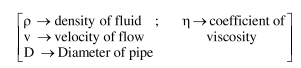# What will be the nature of flow of water from a circular tap,

Question:

What will be the nature of flow of water from a circular tap, when its flow rate increased from $0.18 \mathrm{~L} / \mathrm{min}$ to $0.48 \mathrm{~L} / \mathrm{min}$ ? The radius of the tap and viscosity of water are $0.5 \mathrm{~cm}$ and $10^{-3} \mathrm{~Pa} \mathrm{~s}$, respectively.

(Density of water : $10^{3} \mathrm{~kg} / \mathrm{m}^{3}$ )

3. Remains turbulent flow

Correct Option: , 4

Solution:

The nature of flow is determined by Reynolds Number.

$\mathrm{R}_{\mathrm{e}}=\frac{\rho \mathrm{vD}}{\eta}$From NCERT

If $\mathrm{R}_{\mathrm{e}}<1000$ $\rightarrow$ flow is steady

$1000$\mathrm{R}_{e}>2000 \rightarrow$flow is turbulent$\mathrm{R}_{\text {einitial }}=10^{3} \times \frac{0.18 \times 10^{-3}}{\pi \times\left(0.5 \times 10^{-2}\right)^{2} \times 60} \times \frac{1 \times 10^{-2}}{10^{-3}}=382.16\mathrm{R}_{\text {efinal }}=10^{3} \times \frac{0.48 \times 10^{-3}}{\pi \times\left(0.5 \times 10^{-2}\right)^{2} \times 60} \times \frac{1 \times 10^{-2}}{10^{-3}}=1019.09\$